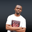Related Tags

php
communitycreator

# How to initialize an empty array in PHPNDUKWE CHIDERA K.

### Overview

An array in PHP is used to hold multiple values that may or may not be of the same data type. In PHP, an array is of three basic types:

• Indexed array
• Associative array
• Multidimensional array

In PHP, arrays are initialized with the array keyword, as shown below:

$myArray = array();  ### How to initialize empty arrays You may ask, “Why do I need to initialize an array without a value?” This is common in PHP codes, where you may want to store the values of a future computation into an array. You basically would declare the array as empty and store the computed values into it. So, how can this be achieved? PHP arrays with no value can be initialized in three ways: 1. $myArray = array();

2. $myArray = []; 3. $myArray = (array) null;

Among the three methods shown, the most common among programmers is the second, which uses []. This is because the second method is marginally faster as it is only a constructor and is just simpler to use.

### Code

Let’s look at some code that uses the techniques listed above.

<?php

$empty1 = (array) null;$empty2 = [];
$empty3 = array(); var_dump($empty1,$empty2,$empty3);

//All above array are empty and will return empty
//pushing values into second type of array
$empty[] ="trails" ;$empty[]  ="trips" ;
$empty[] ="tricks" ;$empty[]  ="trails" ;
var_dump (\$empty) ;
?>

RELATED TAGS

php
communitycreator

CONTRIBUTORNDUKWE CHIDERA K.
RELATED COURSES

View all Courses

Keep Exploring

Learn in-demand tech skills in half the time